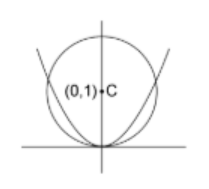Courses
Courses for Kids
Free study material
Free LIVE classes
MoreLIVE
Join Vedantu’s FREE Mastercalss

# Find the range of $'a'$ for which the parabola $y=a{{x}^{2}}$ and the unit circle with centre at $(0,1)$ meet each other at two points other than origin.Verified
361.8k+ views
Hint: To find the range of $'a'$ for which the parabola and the circle intersect each other at points other than origin, we will first find the equation of the circle with the given centre and then substitute the equation of the parabola in the equation of the circle and solve it to get the desired range.

We have a parabola of the form $y=a{{x}^{2}}$ and a unit circle with centre at $(0,1)$.
We want to find the range of $'a'$ for which the two curves will intersect at points other than the origin.
We know that the equation of circle with centre $(h,k)$ and radius $r$ is${{(x-h)}^{2}}+{{(y- k)}^{2}}={{r}^{2}}$.

Substituting $h=0,k=1,r=1$ in the above equation, we get ${{x}^{2}}+{{(y-1)}^{2}}={{1}^{2}}$
$...(1)$ as the equation of our circle.
Now, we have $y=a{{x}^{2}}$ $...(2)$ as the equation of our parabola.
To find the point of intersection of the two curves, we will rewrite the equation of parabola
as $\dfrac{y}{a}={{x}^{2}}$ $...(3)$
We will substitute this equation of parabola in equation $(1)$.
Thus, we get
\begin{align} &\Rightarrow\dfrac{y}{a}+{{(y-1)^{2}}}={{1}^{2}}\\ &\Rightarrow\dfrac{y}{a}+{{y}^{2}}+1-2y=1 \\ &\Rightarrow\dfrac{y}{a}+{{y}^{2}}-2y=0 \end{align}Now factorizing the above equation, we get $y(\dfrac{1}{a}+y-2)=0$.
So, we have
$\Rightarrow y=0,y=2-\dfrac{1}{a}$
Thus, for a point of intersection of two curves other than the origin, we have $y=2-\dfrac{1}{a}$.
Substituting the above value of $y$ in the equation $(3)$, we get ${{x}^{2}}=\dfrac{y}{a}=\dfrac{2}{a}-\dfrac{1}{{{a}^{2}}}$.
As we are excluding the origin from our point of intersection of the two curves, we have ${{x}^{2}}>0$
\begin{align} & \Rightarrow \dfrac{2}{a}-\dfrac{1}{{{a}^{2}}}>0 \\ & \Rightarrow \dfrac{2a-1}{{{a}^{2}}}>0 \\ & \Rightarrow 2a-1>0 \\ & \Rightarrow a>\dfrac{1}{2} \\ \end{align}
$\Rightarrow a\in \left( \dfrac{1}{2},\infty \right)$
Hence, the required range of value of $'a'$ is $a\in \left( \dfrac{1}{2},\infty \right)$.

Note: We can also solve this question by substituting the value of $y$ in terms of $x$ and then solve for $x$. However, that will be time consuming to calculate. Also, one must keep in mind that ${{x}^{2}}>0$ as we are looking for points of intersection of two curves other than the origin.
Last updated date: 24th Sep 2023
Total views: 361.8k
Views today: 11.61k